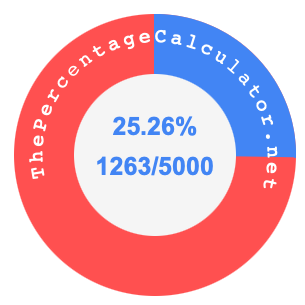25.26 percent as a fractionHere we will not only show you how to convert 25.26 percent to a fraction (25.26% as a fraction), but also illustrate 25.26 percent and 25.26 as a fraction on a pie chart.

Converting 25.26 percent to a fraction means that you want to convert 25.26% to a fraction with a numerator and denominator.

Percent means per hundred, so 25.26 percent means 25.26 per hundred. Thus, you can make 25.26 the numerator and 100 the denominator, and make 25.26 percent a fraction, like this:

25.26/100

The numerator and denominator in a fraction should not have a decimal point. You can eliminate the decimal point by multiplying both the numerator and denominator by 100 to get the following fraction:

2526/10000

The greatest common factor (GCF) of 2526 and 10000 is 2. Therefore, simplify the fraction above by dividing the numerator and deniminator by 2, like this:

2526 ÷ 2= 1263
10000 ÷ 2 = 5000

That's it! 25.26 percent as a fraction in its simplest form is displayed below:

25.26% = 1263/5000

The illustration below shows 25.26 percent and 25.26 as a fraction on a pie chart. As you now know, 25.26% is the same as 1263/5000.Percent to Fraction Converter
Here you can submit another percent that we can convert to a fraction.

25.27 percent as a fraction
Here is the next percent on our list that we converted to a fraction.